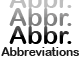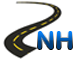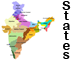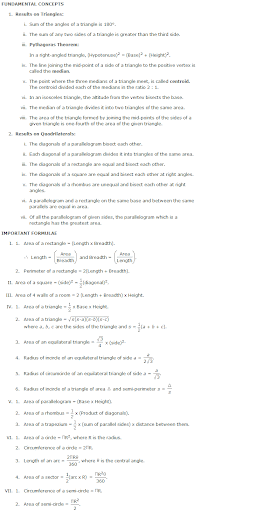Area -List of AbbreviationsNational Highways in IndiaStates & Union Territories of IndiaCurrency of All Countries

# Area

Overview:Sample Questions:

1. The perimeter of a rectangular field is 480 m and the ratio between the length and breadth 5:3. the area of the field is:
A. 12,500m B. 13,500m
C. 14,500m D. 13,000m

Ans: B

2. if the length and breadth of a rectangular plot are increased by 50% and 20% respectively, then the new area is how many time the original area?
A. 1900 B. 1950
C. 1925 D. 1915

Ans: D

3. The length of a rectangle is increased by 60%/ By what percent would the width have to be decreased to maintain the same area?
A. 37.5 B. 38.2
C. 36.5 D. 37.0

Ans: A

4. If each side of a square is increased by 50% the ratio of the area of the resulting square to the area of the given square is ?
A. 5:4 B. 3:4
C. 4:5 D. 4:3

Ans: B

5. The length of a blackboard is 8cm more than its breadth. If the length is increased by 7cm and breadth is decreased by 4cm, the area remains the same. The length and breadth of the black board (in cms) will:
A. 26, 20 B. 28, 24
C. 28, 22 D. 28, 20

Ans: D

6. The cost of carpeting a room 18m long with a carpet 75cm wide at 45 pa per meter is Rs.81. The breadth of the room is:
A. 7.5m B. 3.5m
C. 5.5m D. 9.5m

Ans: A

7. Of the two square fields the area of one is 4 hecture while other other one is broader by 1%. The difference in their ares is:
A. 100m B. 120m
C. 110m D. 115m

Ans: A

8. A hall 20m long and 15m in broad is surrounded by a verandha of uniform width of 2.5 m. the cost of flooring the verandha at Rs.3.50 per square meter:
A. Rs.120 B. Rs.115
C. Rs.100 D. Rs.112

Ans: C

9. The number of marble slabs of size 20cm x 30cm required to pave the floor of a square room of side 3 m is:
A. 120 B. 150
C. 145 D. 130

Ans: B

10. IF each side of a square is increased by 25% its area is increased by:
A. 55.25% B. 55.30%
C. 56.25% D. 55.85%

Ans: C

11. A man walking at the speed of 4 km/ph crosses a square field diagonaly in 3 minutes. The area of the field is:
A. 12,500m B. 13,500m
C. 20,000m D. 13,000m

Ans: C

12. A square and a rectangle have equal areas. If their perimeters are p1 and p2 respectively, then:
A. p1 C. p1>=p2 D. p1>=p2

Ans: A

13. if the perimeters of a square and a rectangle are the same, the areas A and B enclosed by them would satisfy the condition:
A. A
C. A>B D. A>=B

ans: C

14. The ratio of the area of a square to that of the square drawn on its diagonal is:
A. 1:2 B. 1:3
C. 1:4 D. 3:1

Ans: A

15. IF the ratio of the areas of two squares is 9:1 the ratio of the perimeters is:
A. 1:4 B. 3:1
C. 1:3 D. 1:2

Ans: B

16. If the diagonal of a rectangle is 17cm long and the perimeter of the rectangle is 46cm then the area of the rectangle is:
A. 125m B. 130m
C. 120m D. 135m

Ans: C

17. If the perimeter of a rectangle and a square each is equal to 80cm and the difference of their areas is 100sqcm, the sides of the rectangle are:
A. 10m, 25m B. 25m, 20m
C. 30m, 20m D. 30m, 10m

Ans: D

18. Of the two square fields the area of one is 4 hecture while other other one is broader by 1%. The difference in their ares is:
A. 120m B. 130m
C. 140m D. 100m

Ans: D

19. The length of a rectangle is twice its breadth. If its length is decreased by 5cm and breadth is increased by 5cm, the area of the rectangle is increased by 75sqcm. The length of the rectangle is:
A. 50m B. 40m
C. 45m D. 30m

Ans: B

20. The cost of cultivating a square field at the rate of Rs.135 per hectare is Rs.1215. The cost of putting a fence around it at the rate of 75 paise per meter would be:
A. 1250 B. 1100
C. 850 D. 900

Ans: D

21. Within a rectangle garden 10m wide and 20m long, we wish to pave a walk around the borders of uniform width so as to leave an area of 96m2 for flowers. How wide should the walk be:
A. 2m B. 5m
C. 4m D. 3m

Ans: A

22. The cost of carpeting a room 18m long with a carpet 75cm wide at 45 pa per meter is Rs.81. The breadth of the room is:
A. 5.5m B. 8.5m
C. 7.5m D. 4.5m

Ans: C

23. A rectangular lawn 55m by 35m has two roads each 4m wide running in the middle of it , one parallel to length and the other parallel to breadth. The cost of traveling the roads at 75p per sq meter is:
A. 258 B. 260
C. 263 D. 257

Ans: A

24. A rectangular field has dimensions 25m by 15m. Two mutually perpendicular passage, 2m wide have been left in the central part. In rest of the field, grass has been grown. The area under the grass is;
A. 310m B. 294m
C. 300m D. 299m

Ans: D

25. The ratio between the length and breadth of rectangular field is 5:4. If the breadth is 20m less than the length the perimeter of the field is:
A. 350m B. 355m
C. 360m D. 358m

Ans: CClick Here to Find Latest Jobs and Current Affairs

Updated: May 31, 2013 — 10:47 am